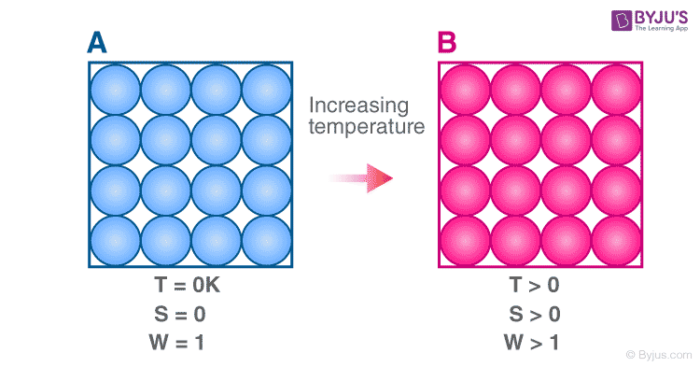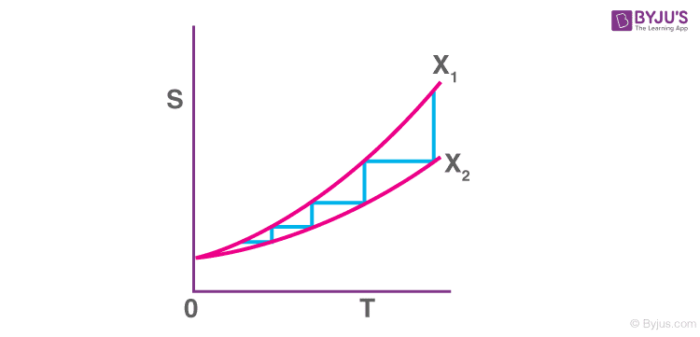# Third Law Of Thermodynamics

## What is the Third Law of Thermodynamics?

The third law of thermodynamics states that the entropy of a perfect crystal at a temperature of zero Kelvin (absolute zero) is equal to zero.

Entropy, denoted by ‘S’, is a measure of the disorder/randomness in a closed system. It is directly related to the number of microstates (a fixed microscopic state that can be occupied by a system) accessible by the system, i.e. the greater the number of microstates the closed system can occupy, the greater its entropy. The microstate in which the energy of the system is at its minimum is called the ground state of the system.

At a temperature of zero Kelvin, the following phenomena can be observed in a closed system:

• The system does not contain any heat.
• All the atoms and molecules in the system are at their lowest energy points.

Therefore, a system at absolute zero has only one accessible microstate – it’s ground state. As per the third law of thermodynamics, the entropy of such a system is exactly zero.This law was developed by the German chemist Walther Nernst between the years 1906 and 1912.

### Alternate Statements of the 3rd Law of Thermodynamics

The Nernst statement of the third law of thermodynamics implies that it is not possible for a process to bring the entropy of a given system to zero in a finite number of operations.

The American physical chemists Merle Randall and Gilbert Lewis stated this law differently: when the entropy of each and every element (in their perfectly crystalline states) is taken as 0 at absolute zero temperature, the entropy of every substance must have a positive, finite value. However, the entropy at absolute zero can be equal to zero, as is the case when a perfect crystal is considered.

The Nernst-Simon statement of the 3rd law of thermodynamics can be written as: for a condensed system undergoing an isothermal process that is reversible in nature, the associated entropy change approaches zero as the associated temperature approaches zero.

Another implication of the third law of thermodynamics is: the exchange of energy between two thermodynamic systems (whose composite constitutes an isolated system) is bounded.

## Why is it Impossible to Achieve a Temperature of Zero Kelvin?

For an isentropic process that reduces the temperature of some substance by modifying some parameter X to bring about a change from ‘X2’ to ‘X1’, an infinite number of steps must be performed in order to cool the substance to zero Kelvin.

This is because the third law of thermodynamics states that the entropy change at absolute zero temperatures is zero. The entropy v/s temperature graph for any isentropic process attempting to cool a substance to absolute zero is illustrated below.From the graph, it can be observed that – the lower the temperature associated with the substance, the greater the number of steps required to cool the substance further. As the temperature approaches zero kelvin, the number of steps required to cool the substance further approaches infinity.

## Mathematical Explanation of the Third Law

As per statistical mechanics, the entropy of a system can be expressed via the following equation:

S – S0 = 𝑘B ln𝛀

Where,

• S is the entropy of the system.
• S0 is the initial entropy.
• 𝑘B denotes the Boltzmann constant.
• 𝛀 refers to the total number of microstates that are consistent with the system’s macroscopic configuration.

Now, for a perfect crystal that has exactly one unique ground state, 𝛀 = 1. Therefore, the equation can be rewritten as follows:

S – S0 = 𝑘B ln(1) = 0 [because ln(1) = 0]

When the initial entropy of the system is selected as zero, the following value of ‘S’ can be obtained:

S – 0 = 0 ⇒ S = 0

Thus, the entropy of a perfect crystal at absolute zero is zero.

## Applications of the Third Law of Thermodynamics

An important application of the third law of thermodynamics is that it helps in the calculation of the absolute entropy of a substance at any temperature ‘T’. These determinations are based on the heat capacity measurements of the substance. For any solid, let S0 be the entropy at 0 K and S be the entropy at T K, then

ΔS = S – S0

$$\begin{array}{l}ΔS = \int^T_0 \frac {C_p dT}{T}\end{array}$$

According to the third law of thermodynamics, S0= 0 at 0 K,

$$\begin{array}{l}S = \int^T_0 \frac{C_p}{T}dT\end{array}$$

The value of this integral can be obtained by plotting the graph of Cp/ T versus T and then finding the area of this curve from 0 to T. The simplified expression for the absolute entropy of a solid at temperature T is as follows:

$$\begin{array}{l}S = \int^T_0 \frac{C_p}{T} dT\end{array}$$

$$\begin{array}{l} S =\int^T_0 C_p d lnT\end{array}$$

= Cp ln T = 2.303 Cp log T

Here Cp is the heat capacity of the substance at constant pressure and this value is assumed to be constant in the range of 0 to T K.

## Frequently Asked Questions – FAQs

Q1

### What is thermodynamics?

Thermodynamics is the branch of physical chemistry that deals with the heat, work, temperature, and energy of the system.

Q2

### What is entropy?

Entropy, denoted by ‘S’, is a measure of the disorder or randomness in a closed system. It is directly related to the number of microstates accessible by the system, i.e. the greater the number of microstates the closed system can occupy, the greater its entropy.

Q3

### What is the first law of thermodynamics?

The first law of thermodynamics states that energy can neither be created nor be destroyed but can be transferred from one form to another.

Q4

### What is the second law of thermodynamics?

The second law of thermodynamics states that the total entropy of an isolated system is increasing continuously.

Q5

### What is the third law of thermodynamics?

The third law of thermodynamics states that the entropy of a system at absolute zero is constant or it is impossible for a process to bring the entropy of a given system to zero in a finite number of operations.